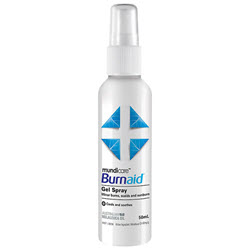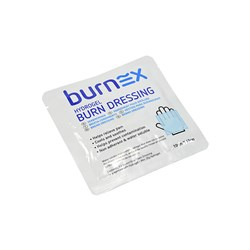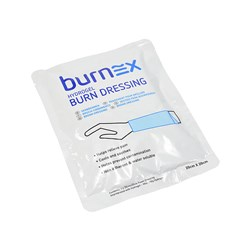# Wound Care

Grid List
\$15.60
Increase value Decrease value
\$3.96
Increase value Decrease value
\$2.17
Increase value Decrease value
\$6.57
Increase value Decrease value
\$10.67
Increase value Decrease value
\$9.91
Increase value Decrease value
\$15.02
Increase value Decrease value
\$12.99
Increase value Decrease value
\$8.48
Increase value Decrease value
\$4.73
Increase value Decrease value
\$1.39
Increase value Decrease value
\$39.85
Increase value Decrease value\$11.74
Increase value Decrease value
\$16.12
Increase value Decrease value
Hydrogen Peroxide 3% 100ml
\$8.27
Increase value Decrease value
\$16.92
Increase value Decrease value
\$14.19
Increase value Decrease value
\$9.20
Increase value Decrease value
\$9.13
Increase value Decrease value
\$45.03
Increase value Decrease value
\$51.55
Increase value Decrease value
\$6.70
Increase value Decrease value
\$38.15
Increase value Decrease value
\$17.45
Increase value Decrease value
\$2.10
Increase value Decrease value
\$2.77
Increase value Decrease value
\$3.03
Increase value Decrease value
\$3.69
Increase value Decrease value
\$7.49
Increase value Decrease value
\$3.48
Increase value Decrease value
\$107.79
Increase value Decrease value
\$193.51
Increase value Decrease value
\$108.99
Increase value Decrease value
\$3.32
Increase value Decrease value
Opsite Post-Op Visable 8x10cm (Box of 20)
\$171.84
Increase value Decrease value
Alco Wipes (200)
In Stock
\$10.62
Increase value Decrease value
\$5.97
Increase value Decrease value
\$3.31
Increase value Decrease value
\$5.04
Increase value Decrease value
\$6.29
Increase value Decrease value
\$7.63
Increase value Decrease value
Combine Roll 9cm x 10m
\$23.08
Increase value Decrease value\$6.50
Increase value Decrease value
\$2.86
Increase value Decrease value\$15.87
Increase value Decrease value
\$4.95
Increase value Decrease value
\$1.29
Increase value Decrease value
\$3.75
Increase value Decrease value
Grid List
##### Products to compare:
Comparing Products